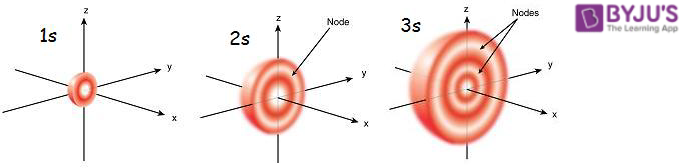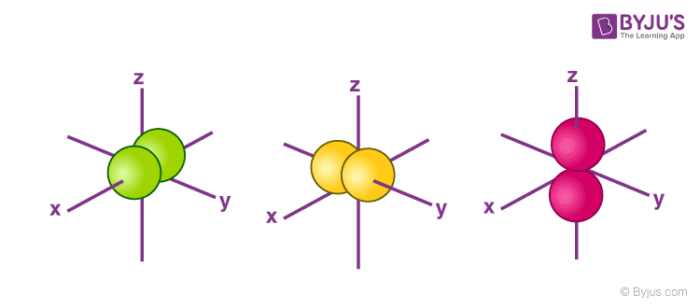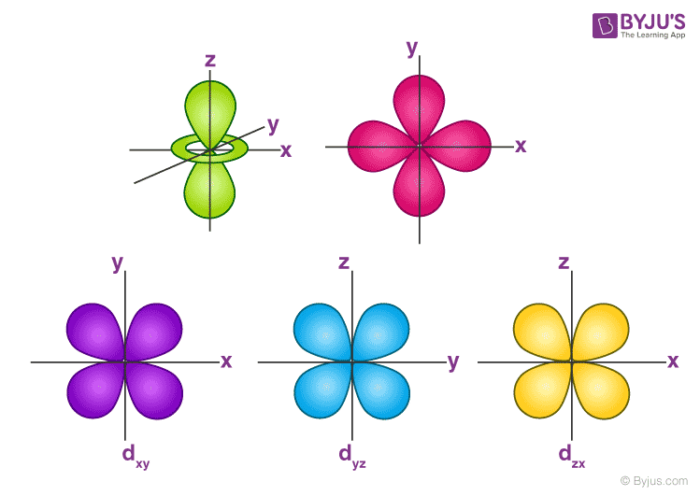# What is Boundary Surface Diagram?

## What is a boundary surface?

Boundary surface diagram is a good diagrammatic representation of the shapes of atomic orbitals. It is the result of the solution of the Schrödinger wave equation.

As we know that the exact position and momentum of an electron cannot be determined (according to the Heisenberg uncertainty principal), we calculate the probability density of finding an electron in a particular region. Boundary surface diagram is a boundary surface or a contour surface drawn in a space for an orbital on which the value of probability density |ψ|2 is constant. The boundary surface diagram of constant probability density is considered a good and acceptable approximation of the shape of an orbital if the boundary surface encloses the region or volume with a probability density of more than 90%. This means that the boundary surface enclosing a constant probability density of, let’s say, 50% won’t be considered good.

## Table of Content

### Why the boundary surface diagram is not taken with constant probability density of 100%?

The answer is at any distance from the nucleus, the probability density of finding an electron is never zero. It will always have some finite value, so it is not possible to draw a boundary surface diagram which encloses a region with 100% probability density.

## Feature of boundary surface diagram

The orbital wave function is represented by the product of radial and angular wave functionThe orbital wave function |ψ|2 means the electron probability density at a point in an atom. The regions where this probability density function is zero are called nodal surfaces or nodes. The number of radial nodes is (n – l – 1). The number of angular nodes is l

1. Shape of the surface diagram:
The boundary surface diagram of an orbital is independent of the principal quantum number.
For e.g.: The boundary surface diagram of s orbital is spherical, so it will be spherical for 1s, 2s, 3s and 4s or for any general ns. The shape doesn’t depend on the principal quantum number (n).
1. Size of the surface diagram:
The boundary surface diagram of an orbital increase in size or volume with an increase in principal quantum number (n).
1. Nodes in the surface diagram
Nodes are the region with very low probability density typically which goes to zero. There are (n-1) nodes in the boundary surface diagram of s-orbital with ‘n’ principal quantum number. This kind of nodes is also observed in the surface diagram of p, d and f orbitals.

## Boundary surface diagram for ‘s’- orbital

• The angular node of ‘s’ orbital is 0. Hence the probability of finding the  electron at a given distance is a constant value.
• So All the s -orbitals are in Spherical shape.
• The radial node of 2s- orbital is  (n – l – 1) = 2-0-1 = 1. So between 1s and 2s there is a region where the probability of the electrons is zero.
• The size of the s orbital increases with increase in n, that is, 4s > 3s > 2s > 1s and the electron is located further away from the nucleus as the principal quantum number increases.## Boundary surface diagram for ‘p’- orbital

• The angular node of the ‘p’ orbital is 1. Hence the probability density function is zero on the plane where the two lobes touch each other.
• So each p orbital consists of two sections called lobes that are on either side of the plane that passes through the nucleus.
• It has three possible orientations.
• All the p orbitals are identical in size, shape and energy but the orientations are different along the x, y and z axes.
• They are designated by  px , py and pz .
• The size of the p orbital increases with increase in n, that is, 5p > 4p > 3p > 2p## Boundary surface diagram for ‘d’- orbital

• The angular node of the ‘d’ orbital is 2.
• It has five possible orientations.
• All five d- orbitals have the same energy but different orientations.
• They are designated by  dxy , dyz , dzx , dx2-y2 , dz2 .Q1

### Why do we not draw a boundary surface diagram?

We don’t draw a boundary surface diagram of orbitals at constant probability because at any distance from the nucleus, the probability density of finding an electron is never zero.

Q2

### How many nodes are present in the 3s orbital?

In s orbital there is no angular node, only radial node is present. The number of radial nodes is (n – l – 1) = 3- 0 -1= 2. So there are 2 nodes present in the 3s orbital.

Q3

### What are radial nodes and angular nodes?

The regions where this probability density function is zero are called nodal surfaces or nodes. Radial node is the region where the probability of finding electrons in the radial wave function is zero; the number of radial nodes is calculated by using formula ‘n – l – 1’. Similarly for angular nodes, the region where the probability of finding electrons for angular wave function is zero; the number of angular nodes is calculated by using formula ‘l’.

Q4

### How many radial nodes are in a 2p orbital?

There are zero radial nodes present in the 2p orbital. The number of radial nodes is (n – l – 1) = 2-1-1 =0..

Q5

### How many nodes are present in a 4p orbital?

In 4p orbital; the number of radial nodes is equal to (n – l – 1) = 4 – 1 -1 =2.
The number of angular nodes is equal to l = 1 . Total of 3 orbitals are present’ in the 4p orbital.

Test your knowledge on boundary surface diagram